# decimal notation

[Joe Monzo]

A notation invented by Graham Breed for representing the MIRACLE family of tunings.

The notation is based on the fact that the MIRACLE generator produces 10- and 11-tone MOS's in which the 11th tone is audibly quite close to the origin tone.

Graham's own webpages describe the decimal notation itself, and the decimal lattices which use it.

Below is an explanatory elaboration based on a post I sent to the tuning list:

> ----- Original Message -----
> From: Joe Pehrson
> To: Yahoo tuning group
> Sent: Thursday, June 21, 2001 6:11 AM
> Subject: [tuning] Re: updated "MIRACLE" definition webpage

> > > > Now, could I please ask you to elaborate a bit on Graham Breed's
> > decimal notation, so I can understand it??

Here's a real quick stab at it, Joe... but it should help.

First of all, realize that Graham's use of numbers here is exactly equivalent to the way we currently use the first seven letters of the alphabet to describe the 7-tone diatonic cardinality. So the digits 0 ... 9 are the 10-tone cardinality of the basic MIRACLE scale.

Secondly, realize that Graham's notation is generalized in that it is applicable to 31-edo and 41-edo as well as 72-edo. I'm going to discuss it here only in terms of 72-edo.

OK, now look at the graph of the 11-tone MIRACLE scale (the one with the green line) on my MIRACLE definition webpage. Keep this window open or print it out... you'll be using my two recent graphs a lot in following what I say here.

Now look at the "melodic" description of Graham's notation in the first section of his webpage.

Let's start the scale at the -5 generator, giving the pitch which is 6&1/6 Semitones, which we will call "0". We are considering Graham's 10-tone scale here, not the 11-tone one I illustrate. So here the +5 generator, giving the pitch at 5&5/6 Semitones, which is the one close to the origin, will be omitted. So we'll use as our bounding notes the -5 and +4 generators.

Here's a list of the basic 10-tone MIRACLE scale, in descending order (as usual with me... sorry Dave Keenan...), with Graham's "s" and "q" interval-spacing:

```Generator Graham's  Semitones          interval between
"note"                 Semitones  72-EDO-degrees

-5        0        6 1/6
>   1 1/2    9    s+q
+4        9        4 2/3
>   1 1/6    7    s
+3        8        3 1/2
>   1 1/6    7    s
+2        7        2 1/3
>   1 1/6    7    s
+1        6        1 1/6
>   1 1/6    7    s
0        5        0
>   1 1/6    7    s
-1        4        10 5/6
>   1 1/6    7    s
-2        3        9 2/3
>   1 1/6    7    s
-3        2        8 1/2
>   1 1/6    7    s
-4        1        7 1/3
>   1 1/6    7    s
-5        0        6 1/6
```

So as Graham explains, "s" in 72-EDO MIRACLE is 2(7/72), and "q" is the new interval we find at 11 tones, which is 2(2/72). So the large interval at the end of the 10-tone cycle is "s+q" = 2(9/72).

Graham's notation uses "v" and "^" as accidentals, in which "the ^ and v symbols denote upward and downward shifts of q".

So if we decide to include that 11th tone of 5 5/6 Semitones, which is the +5 generator, it would be called 0v, which indicates that the origin, 6 1/6Semitones, has been flattened by "q", which is 2(2/72) = 33 1/3 cents = 1/3 Semitone = "1/6-tone":

```      "0"       -     "q"      = "0v"
6 1/6 Semitones - 1/3 Semitone = 5 5/6Semitones
```

Here are my graphs of Graham's notation, for both the 11-tone and 32-tone MIRACLE cycles: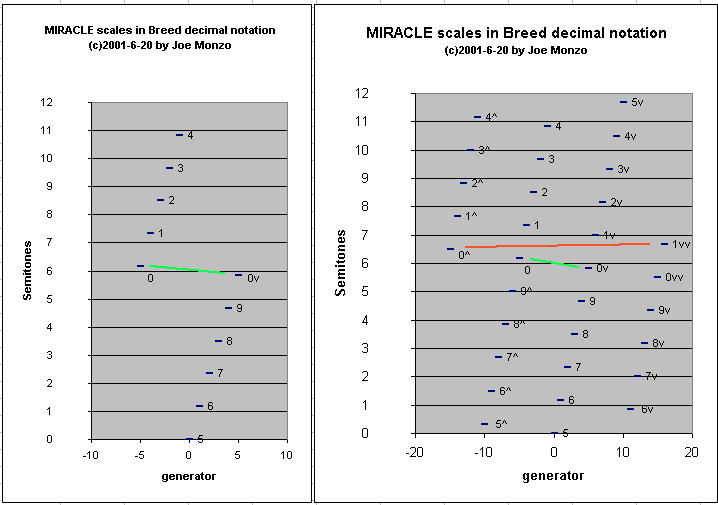So now look at the 32-tone MIRACLE graph, and notice how all subsequent pitches added to our gamut will be described in terms of the basic 10-tone scale tabulated above.

So if we continue the cycle on the positive side, adding the +6 generator = 7 Semitones, it would be "1v", which is:

```      "1"       -     "q"      = "1v"
7 1/3 Semitones -  1/3 Semitone = 7 Semitones
```

and so on.

If we continue on the negative side, adding the -6 generator = 5 Semitones, it would be "9^", which is:

```      "9"       +     "q"      = "9^"
4 2/3 Semitones +  1/3 Semitone = 5 Semitones
```

and so on.

The -15 generator = 6 1/2 Semitones would be "0^", because it is one "q" higher than our origin note "0":

```      "0"       +     "q"      = "0^"
6 1/6 Semitones +  1/3 Semitone = 6 1/2 Semitones
```

The -16 generator would be "9^^", because it is one "q" higher than "9^".

On the positive side, the +15 generator = 5&1/2 Semitones would be "0vv", because it is one "q" lower than "0v":

```      "0v"      -     "q"      = "0vv"
5 5/6 Semitones -  1/3 Semitone = 5 1/2 Semitones
```

The +16 generator = 6 2/3 Semitones would be "1vv", because it is one "q" lower than "1v":

```      "1v"      -     "q"      = "1vv"
7 Semitones -  1/3 Semitone = 6 2/3 Semitones
```

Note, however, that this "1vv" is 1/2 "q" higher than "0^". We've run up against the next level of enharmonicity, which delimits the Canasta scale.

Below is a graph of the entire 72-EDO superset in Graham's decimal notation. I've used a different starting point for the decimal nominals here, calling the 0 generator also the 0 nominal, to conform with the usage on Graham's webpage. A purple line connects the -31 and +41 generators, which give exactly the same pitch, thus closing the cycle at 72 tones. 9^^^^ and 1vvvv are thus enharmonically equivalent in this notation.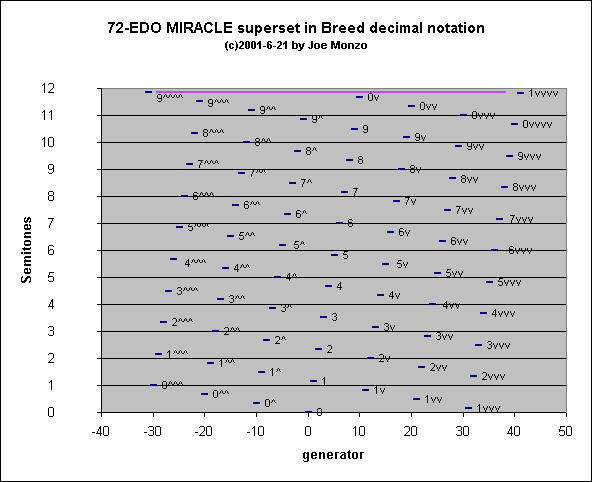Below is an illustration of the 21-tone blackjack subset of 72edo notated in my 4-line-staff adaptation of Graham's decimal notation: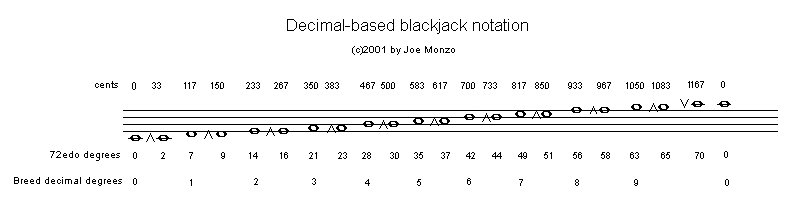And below is a score of Graham's implied-7-limit blackjack "comma-pump" chord progression, notated in the same adapted decimal notation (click on the graphic to hear an mp3 of the chord progression):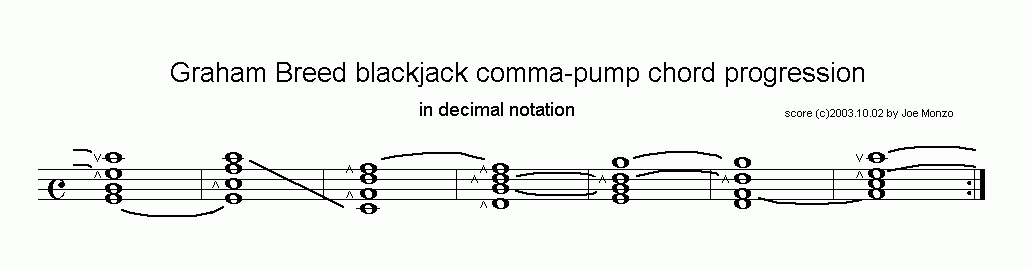Below is the same chord-progression, this time notated in the 72-edo version of HEWM notation, for comparison: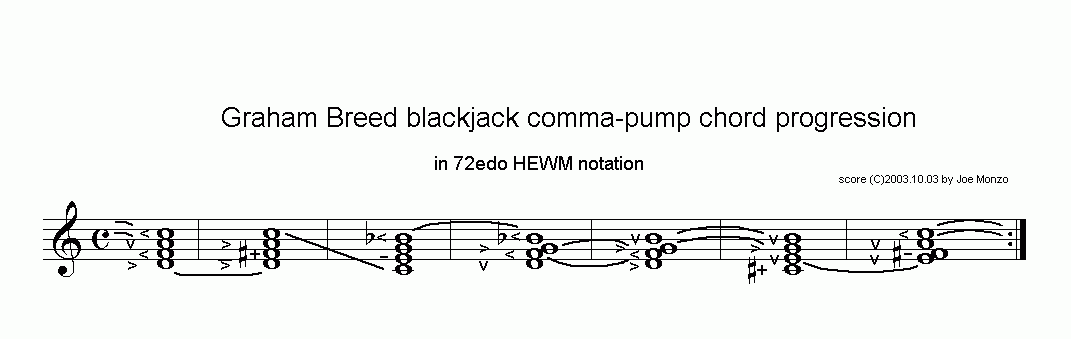. . . . . . . . .

The tonalsoft.com website is almost entirely the work of one person: me, Joe Monzo. Please reward me for my knowledge and effort by choosing your preferred level of financial support. Thank you.

 support level donor: \$5 USD friend: \$25 USD patron: \$50 USD savior: \$100 USD angel of tuning: \$500 USD microtonal god: \$1000 USD